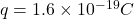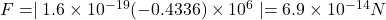## An electron that has a velocity with x component 2.6 × 106 m/s and y component 2.4 × 106 m/s moves through a uniform magnetic field with x c

Question

An electron that has a velocity with x component 2.6 × 106 m/s and y component 2.4 × 106 m/s moves through a uniform magnetic field with x component 0.029 T and y component -0.14 T. (a) Find the magnitude of the magnetic force on the electron. (b) Repeat your calculation for a proton having the same velocity.

in progress 0
1 month 2021-08-06T14:43:03+00:00 1 Answers 6 views 0

We are given that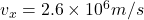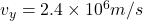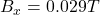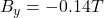a.We have to find the magnitude of the magnetic force on the electron.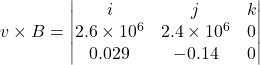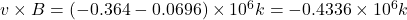Charge on an electron,q=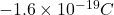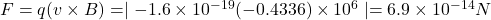Force act along positive z- direction.

b.Charge on proton=